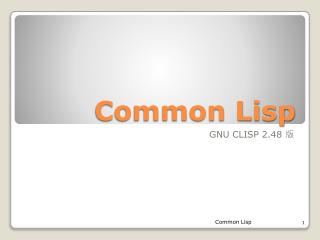Download PresentationCommon Lisp# Common Lisp - PowerPoint PPT Presentation

Download Presentation##### Common Lisp

Download Policy: Content on the Website is provided to you AS IS for your information and personal use and may not be sold / licensed / shared on other websites without getting consent from its author. While downloading, if for some reason you are not able to download a presentation, the publisher may have deleted the file from their server.

- - - - - - - - - - - - - - - - - - - - - - - - - - - E N D - - - - - - - - - - - - - - - - - - - - - - - - - - -
##### Presentation Transcript

1. Common Lisp GNUCLISP 2.48 版 Common Lisp

2. Symbols(符號) • symbol 就是一串字元，你可以在符號中包含英文字母、數字、連接符號來混合產生 symbol。 • 如果你只有使用數字，並且至多在拿減號當起始字元:(-5)，那將會被 Lisp 視為是數字，而不是 symbol 。 • 下面是 symbol 的一些範例： • a 、b、c1、d123、math-227 Common Lisp

3. Symbols(符號) • 你可以像下面的例子一樣的使用 symbol 。 • （在 > 提示符號後面的就是你的輸入給 Lisp 直譯器的內容，而其他的就是 Lisp 直譯器所送回來輸出的結果。而 ";" 分號則是 Lisp 的註解符號，在分號之後到該行結束的資料都會被直譯器所忽略。） >(setq a 5) ;把數值 5 存入 a 這個 symbol 裡面。 5 > a ; 取得 a 這個 symbol 所存的值。 5 Common Lisp

4. Numbers(數值) • 整數型別的定義就是一連串的數字，並且最前方可以選擇性的加上(+ - * /)。而實數包含有整數，而且還可以有小數點，或是也可以用科學記號表示。有理數則是兩個整數相除而得，也就是在兩個整數中間加上 / 。 • Lisp 還支援複數型別，利用像是 #c(r i) 這樣表示複數，其中 r 表示複數的實部，i表示複數的虛部。上列的任何一種都稱做是 Number (數字)型別。 • 下面是一些 Number 型別的例子： 5、+17、-34、3/4、3.1415、1.722e-15 #c(1.722e-15 0.75) Common Lisp

5. Numbers(數值) • 對於數字可以做的運算，一些常見的算數函數如 +, -, *, /,gcd, mod, sin, cos, sqrt, exp ,expt等等都有內建，而且這些內建的算數函數可以接受任何 Number 型別的參數。 +, -, *, / 這四的函數的傳回值型別會隨輸入參數的型別而自動延伸為較廣的型別範圍，比如說 • 整數加上有理數的傳回值，就會是範圍較廣的有理數； • 而有理數加上實數的傳回值，是實數； • 實數加上複數的傳回值，則是複數。 • 下面是一些例子： Common Lisp

6. > (+ 3 3/4) ;傳回值型別範圍自動加廣 15/4 > (exp 1) ;自然對數的基底 e ，1為次方數 2.7182817 > (expt 3 4.2) ;指數函數，以 3 為底數，次方數 100.90418 是 4.2 >(+ 5 6 7 8) ;內建的 +,-,*,/ 四個算數函數都 可以接受多個參數值的呼叫 >(sqrt 100) ; 對100開根號 10 Common Lisp

7. Function (函數) • 函數用數學表達式都是前綴表達式的形式。 >(+a 6);把 a這個symbol的值當作是加法函數的參數 11 >(*a 3);把 a這個symbol的值當作是乘法函數的參數 15 (+123 4 5 678910) ; 加法函數可以接受 55任意多個輸入參數 >(+321(* 1 2 3 )) ;括號內 1, 2, 3 作乘法運 12 算,再與3,2,1 作加法運算 Common Lisp

8. Define function (定義函數) • 定義函數: define function => defun • 函數名稱: 自訂的 symbol • 函數參數:(n), (x), (c1), . . . • 函數的運算:(+n 1) , (* x x), . . . >(定義函數 函數名稱 (參數)(運算)) >(defun square (x) (* x x ) ) ;定義一個叫做 square 的函數,參數是 x, 運算是將 x 乘上 x , 即 x2 . SQUARE ;Lisp 直譯器會顯示定義的函數 Common Lisp

9. Define function (定義函數) • (函數名稱 輸入參數 ) >(square 5 ) ;對函數 square 輸入 5 作運算 25;傳回結果 >(defun two (x y) (+ x y 10) ) ;定義 two 此函數，有兩個變數 x ,y, 作加法運算。 TWO >(two 1 9 ) ;對函數 two 輸入兩變數的參數值 19 20;傳回結果 Common Lisp

10. Function (函數)--n! • Algorithm 1A Recursive Algorithm for Computing n! (section 4.4 p.312) • procedurefactorial (n: nonnegative integers) if n=0 thenfactorial(n) :=1 elsefactorial(n) := n．factorial(n-1) >(defun fact (x) (cond((= x 0 ) 1) ;定義 fact(0)=1 (t (* x (fact (- x 1)))));定義 fact(x)=x*fact(n-1) • ;cond相當於 if …then 的用法, 類似於 C 語言中的 switch，t 表示真,函數體 的最後 一個 form 作為返回值返回。 Common Lisp

11. Function (函數) • Lisp 中呈現如圖: • (fact 5) 表示5!,當輸入參數為 -1,則超出範圍須重設參數值。 Common Lisp

12. Function (函數)-- gcd • Algorithm 4A Recursive Algorithm for Computing gcd(a ,b). (section 4.4 p.313) • procedure gcd(a, b: nonnegative integers with a <b) if a =0 thengcd(a ,b):= b else gcd(a, b) := gcd(b mod a , a ) • mod(餘數) >(mod 12 5) 2 • gcd (最大公因數) >(gcd 12 27) 3 Common Lisp

13. Function (函數) > (defunggcd (a b) (cond ((= a 0) b) ;定義 gcd(0,b)=b (t (ggcd (mod b a) a)))) ;定義 gcd(a, b) = gcd(b mod a, a) Common Lisp

14. Fibonacci function (函數) • Algorithm 7A Recursive Algorithm for Fibonacci Numbers. (section 4.4 p.316) procedurefibonacci (n: nonnegative integers) if n=0 thenfibonacci(0) :=0 else if n=1 thenfibonacci(1) :=1 elsefibonacci(n) := fibonacci(n-1) + fibonacci(n-2) Common Lisp

15. Fibonacci function (函數) • 根據定義可以很方便的得到一個求f(n) 的演算法： >(defunfibonacci (n) (cond ((= n 0) 0) ;定義 f(0)=0 ((= n 1) 1) ;定義 f(1)=1 (t (+ (fibonacci (- n 1)) (fibonacci (- n 2 )))))) ;定義 f(n) := f(n-1) + f(n-2) Common Lisp

16. Fibonacci function (函數) • Lisp 結果呈現如圖: • (fibonacci 10)=55 ,當輸入參數為 -1,則超出範圍須重設參數值。 Common Lisp

17. 參考資料: • http://math.pro/db/thread-68-1-1.html • http://wiki.ubuntu.org.cn/index.php?title=Common_LISP_Hints&variant=zh-tw • Freesourse http://sourceforge.net/projects/clisp/ GNU LISP 2.48 版本 Common Lisp

18. Q & A Thanks for your listening. Common Lisp